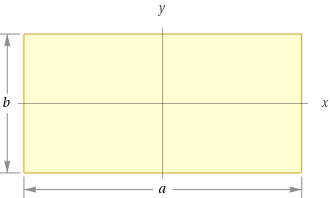Search IntMath
Close

450+ Math Lessons written by Math Professors and Teachers

5 Million+ Students Helped Each Year

1200+ Articles Written by Math Educators and Enthusiasts

Simplifying and Teaching Math for Over 23 Years

# Rectangle in Geometry## What is a Rectangle?

A rectangle is a four-sided shape with four right angles. The opposite sides are equal in length and the interior angles are all 90 degrees. Rectangles are classified as quadrilaterals and two-dimensional shapes. Quadrilaterals are geometric shapes that consist of four sides, and two-dimensional shapes are flat figures that have length and width, but no thickness.

Rectangles are also classified as polygons, which are shapes with three or more sides. The sum of the interior angles in a rectangle is 360 degrees. Rectangles can be further broken down into two categories: squares and rectangles with unequal sides.

## Properties of Rectangles

Rectangles have four sides, four vertices, and four angles. The opposite sides are equal in length and the opposite angles are equal in measure. The diagonals of a rectangle are congruent, or equal in length, and bisect each other. The area of a rectangle is the product of its length and width.

The perimeter of a rectangle is equal to the sum of the lengths of the four sides. The formula for the perimeter of a rectangle is P = 2l + 2w, where l is the length and w is the width of the rectangle.

## Examples of Rectangles

Rectangles can be found in many everyday objects, such as books, notebooks, doors, windows, and televisions. In nature, rectangles can be found in the shape of clouds, leaves, and even snowflakes. Rectangles can also be found in artwork and architecture.

## Practice Problems

1. Find the perimeter of a rectangle with a length of 10 feet and a width of 4 feet.

Answer: The perimeter of the rectangle is 28 feet.

2. Find the area of a rectangle with a length of 8 inches and a width of 3 inches.

Answer: The area of the rectangle is 24 square inches.

3. What is the sum of the interior angles of a rectangle?

Answer: The sum of the interior angles of a rectangle is 360 degrees.

4. Find the length of the diagonal of a rectangle with a length of 8 inches and a width of 6 inches.

Answer: The length of the diagonal is 10 inches.

5. Find the perimeter of a rectangle with a length of 15 feet and a width of 10 feet.

Answer: The perimeter of the rectangle is 50 feet.

6. Find the area of a rectangle with a length of 12 inches and a width of 8 inches.

Answer: The area of the rectangle is 96 square inches.

## Summary

In geometry, a rectangle is a four-sided shape with four right angles. The opposite sides are equal in length and the interior angles are all 90 degrees. Rectangles are classified as quadrilaterals, two-dimensional shapes, and polygons. The sum of the interior angles in a rectangle is 360 degrees. The area of a rectangle is the product of its length and width and the perimeter of a rectangle is equal to the sum of the lengths of its four sides. Rectangles can be found in many everyday objects and in nature.

## FAQ

### What is the definition of a rectangle?

A rectangle is a four-sided shape with opposite sides that are equal in length and four right angles.

### What are the properties of a rectangle?

A rectangle has four sides and four angles, all of which are right angles (90�). The opposite sides of a rectangle are equal in length and the opposite angles are equal in measure. The diagonals of a rectangle have the same length and bisect each other.